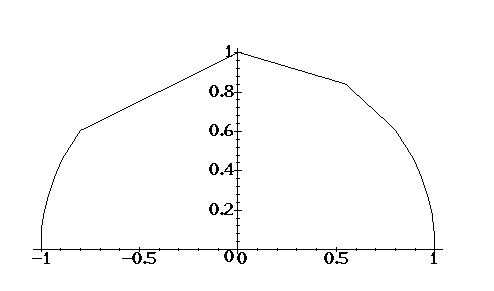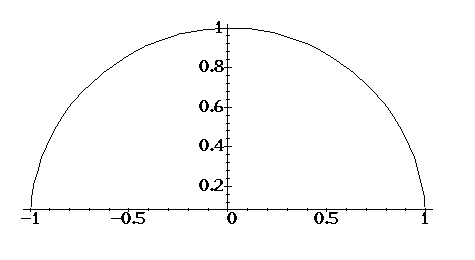## Plotting in Maple: 2d graphics Parametric Plots

Maple can plot curves described parametrically. Probably the best known parametric equation is the circle, which can be described by

x=cos(t)    y=sin(t)    0<t<2Pi .
```
plot( [cos(t), sin(t), t=0..2*Pi] );
```We can, of course, readily increase the radius by multiplying both coordinates by a constant, and move the center by adding a constant term. Thus, a circle of radius 2 and center at (1,0) is given by

```plot( [2*cos(t) + 1, 2*sin(t), t=0..2*Pi] );
```Just as we did when we wanted to the graphs of more than one function on the same axes, we can plot the graphs of more than one parametric function by representing them as a set. Thus, a pair of circles of radius 2 is given by

```
plot( {[2*cos(t) + 1, 2*sin(t), t=0..2*Pi],
[2*cos(t) - 1, 2*sin(t), t=0..2*Pi]},
scaling=constrained);
```Note that we explicitly stated that we wanted the scaling to be constrained, so that Maple wouldn't distort our circles to look like ellipses.

One thing worth keeping in mind is that Maple needs to sample the domain in order to produce the plot; thus, some parameterizations produce more accurate plots than others. For example, if we chose to parameterize a semicircle in the following strange way, we wouldn't get a very good picture:

```plot([sin(arctan(t)),cos(arctan(t)),t=-1000..1000],
scaling=constrained);
```This is because most of the t-values correspond to the beginning and end of the range; Maple only samples 3 or 4 values that correspond to angles between Pi/4 and 3Pi/4.

Changing things slightly makes for a much nicer picture:

```plot([sin(v),cos(v),v=tan(-1000)..tan(1000)],
scaling=constrained);
```(Of course, this whole example is silly... you'd never parameterize a circle like this at all. I just wanted to make a point).